# Radial and elliptical profiles of Image DataSherpa Threads (CIAO 4.13 Sherpa v1)

## Overview

#### Synopsis:

This thread shows how you can use the sherpa_contrib package—part of the CIAO contributed scripts—to plot radial or elliptical profiles of your imaging fits. These can be used to visualize the fit results, and help you interpret the results—e.g. to see how well the model represents the data or to let you fine tune model parameters before a fit to try and reduce the fit time. The thread is based on the Fitting Imaging Data thread, which should be read before this thread.

Last Update: 11 Dec 2019 - Updated for CIAO 4.12, using Matplotlib rather than ChIPS.

## Create and Read Fits Image Data

unix% download_chandra_obsid 1838

This repeats the data creation step of the imaging thread, which should be read for an explanation of the steps.

unix% punlearn dmcopy
unix% dmcopy \
"acisf01838_repro_evt2.fits[sky=box(4059.25,4235.75,521.5,431.5)][bin x=::2,y=::2]" \
image2.fits


After starting Sherpa, we load in both the radial-profile code and the utility routines, then the data (the from .. import line only has to be called once per session):

sherpa> from sherpa_contrib.all import *


An error message of

ImportError: No module named sherpa_contrib


means that the CIAO contributed scripts package has not been installed or is out of date.

We then set up the coordinate system we use for the fit, along with the statistic and optimization method:

sherpa> set_coord("physical")
sherpa> set_stat("cash")
sherpa> set_method("simplex")


We can review the current choices for these settings using the following commands or with the show_method and show_stat commands:

sherpa> get_coord()
'physical'
sherpa> get_stat_name()
'cash'
sherpa> get_method_name()


We finish this section by restricting the region to be fitted:

sherpa> notice2d("circle(4072.46,4249.34,108)")


## Initial fit to the data: Gaussian plus constant

We start with a single Gaussian component to represent the bulk of the emission, and restrict the range of the parameters to values appropriate to this dataset. We use values somewhat more restrictive than used in the original thread, the component name src rather than g1, and take advantage of the guess routine to set sensible limits on the parameter values:

sherpa> set_source(gauss2d.src)
sherpa> print(src)
gauss2d.src
Param        Type          Value          Min          Max      Units
-----        ----          -----          ---          ---      -----
src.fwhm     thawed           10  1.17549e-38  3.40282e+38
src.xpos     thawed            0 -3.40282e+38  3.40282e+38
src.ypos     thawed            0 -3.40282e+38  3.40282e+38
src.ellip    frozen            0            0        0.999
src.theta    frozen            0     -6.28319      6.28319    radians
src.ampl     thawed            1 -3.40282e+38  3.40282e+38

sherpa> guess(src)
sherpa> print(src)
gauss2d.src
Param        Type          Value          Min          Max      Units
-----        ----          -----          ---          ---      -----
src.fwhm     thawed           10  1.17549e-38  3.40282e+38
src.xpos     thawed       4069.5       3965.5       4179.5
src.ypos     thawed       4250.5       4142.5       4356.5
src.ellip    frozen            0            0        0.999
src.theta    frozen            0     -6.28319      6.28319    radians
src.ampl     thawed          263        0.263       263000

sherpa> set_par(src.fwhm, min=0.1, max=300, val=20)
sherpa> set_par(src.ampl, min=0.1, max=1000, val=20)


The last two sets of lines could also have been written:

sherpa> src.fwhm = 20
sherpa> src.fwhm.min = 0.1
sherpa> src.fwhm.max = 300

sherpa> src.ampl = 20
sherpa> src.ampl.min = 0.1
sherpa> src.ampl.max = 1000


The current source model definition may be displayed:

sherpa> show_model()
Model: 1
gauss2d.src
Param        Type          Value          Min          Max      Units
-----        ----          -----          ---          ---      -----
src.fwhm     thawed           20          0.1          300
src.xpos     thawed       4069.5       3965.5       4179.5
src.ypos     thawed       4250.5       4142.5       4356.5
src.ellip    frozen            0            0        0.999
src.theta    frozen            0     -6.28319      6.28319    radians
src.ampl     thawed           20          0.1         1000


We now create our first radial plot of the data using the prof_data command:

sherpa> prof_data()
sherpa> plt.xscale('log')
sherpa> plt.yscale('log')


The resulting plot is shown in Figure 1:

### Figure 1: Initial radial profile of the data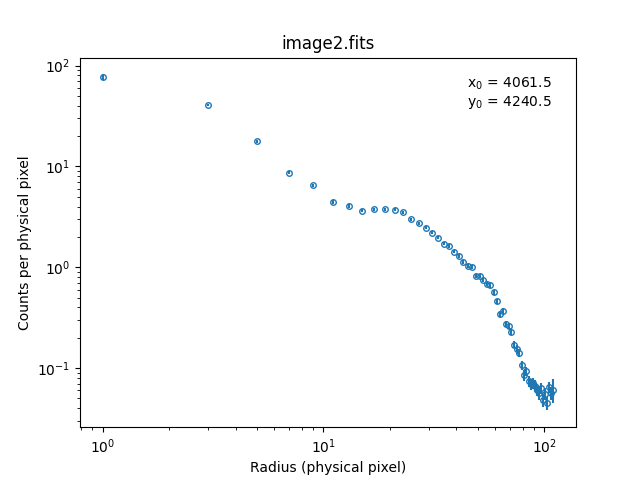### Figure 1: Initial radial profile of the data

The plot shows the radial profile of the data, using the current model values to define the profile center: in this case src.xpos and src.ypos. These values are displayed in the top-right of the plot as x0 and y0. The profile shows that a single component is unlikely to be sufficient to adequately describe the profile.

As no arguments were given to prof_data, the profile was generated using the bin width of the data (so in this case 2 physical pixels), and extends from $$r=0$$ to the maximum radius of the data (in this case close to 110 sky pixels).

Note that the errors are calculated using the equation $$\mathrm{error} = 1 + \sqrt{N + 0.75}$$ where $$N$$ is the number of counts in a bin (and then normalized by the bin area).

We decide to include a background component before fitting, and so add in a constant model to the source expression. The value of the background model was estimated from Figure 1; this suggests a level of 0.05 counts per physical pixel. However, since the data has been binned by 2 in each direction (the cdelt field is 2), then we need to use a value of $$2 \times 2 \times 0.05$$, as shown below:

sherpa> print(get_data().sky)
physical
crval    = [3798.5,4019.5]
crpix    = [0.5,0.5]
cdelt    = [2.,2.]

sherpa> set_source(src + const2d.bgnd)
sherpa> bgnd.c0 = 0.2
sherpa> bgnd.c0.max = 100


The prof_fit command will produce a radial profile of the fit overlain on the data (similar to how plot_fit works). Before making this call we change the plot preferences so that both axes are drawn with log-scaling. We use the optional label argument of prof_fit to turn off display of the labels that show the profile center. The result is Figure 2.

sherpa> get_data_prof_prefs()["xlog"] = True
sherpa> get_data_prof_prefs()["ylog"] = True
sherpa> prof_fit(label=False)


### Figure 2: Initial radial profile of the model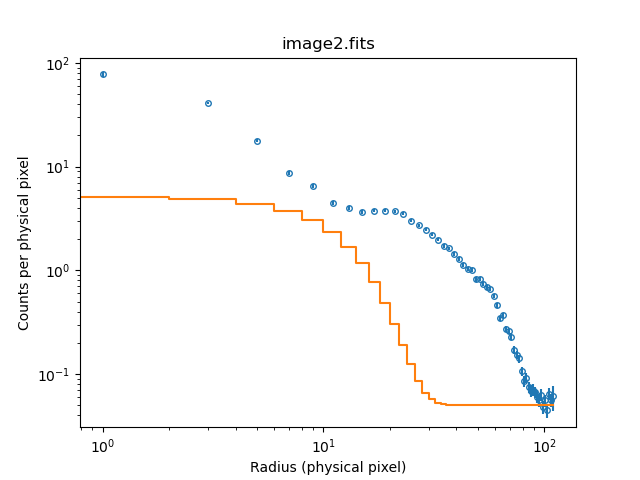### Figure 2: Initial radial profile of the model

The red line shows the radial profile of the current model. Since there has been no fit, the model does not describe the emission very well.

As the label argument was set to False, no labels appear in the top-right of this plot.

It is obvious, from Figure 2, that the width of the Gaussian is too small. A value roughly twice the current size would be a better fit, so we change the src.fwhm value before our first fit.

sherpa> src.fwhm = src.fwhm.val * 2
sherpa> fit()
Dataset               = 1
Statistic             = cash
Initial fit statistic = -30661.2
Final fit statistic   = -48907.8 at function evaluation 527
Data points           = 9171
Degrees of freedom    = 9166
Change in statistic   = 18246.6
src.fwhm       57.9477
src.xpos       4070.4
src.ypos       4251.11
src.ampl       23.3562
bgnd.c0        0.266365


Note that we had to say "src.fwhm.val * 2" rather than "src.fwhm * 2" in the assignment operator. If we did not include the trailing ".val" to get at the numeric value of the parameter, Sherpa would have tried to create a parameter link to itself, which would have failed with the following error message:

ParameterError: requested parameter link creates a cyclic reference


We now use the prof_fit_resid and image_fit commands to see how well the model fits the data. The output of the prof_fit_resid command is shown in Figure 3.

sherpa> image_fit()
sherpa> prof_fit_resid()
sherpa> plt.savefig('fit.png')


### Figure 3: A Gaussian plus constant fit to the data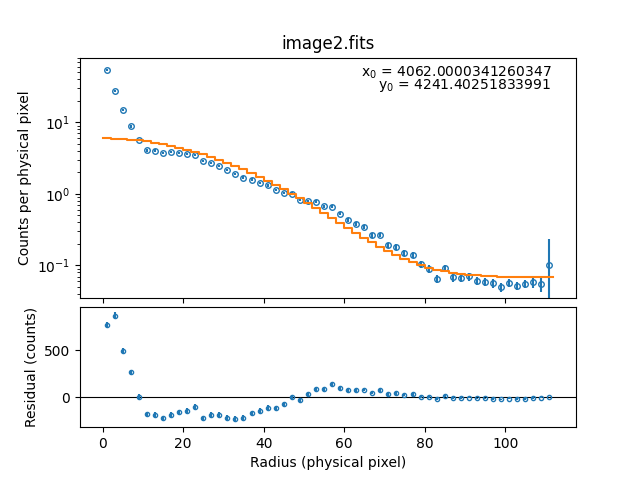### Figure 3: A Gaussian plus constant fit to the data

The top plot shows the data (circles) and best-fit model (red line), whilst the bottom plot shows the residuals—measured as (data - model)—of this fit. Note that the residuals are not normalized by the area of each annulus.

The y-axis of the top plot in Figure 3 is drawn with a logarithmic-scale since we earlier set the data plot preference for ylog to True. The x-axis has remained with a linear-scale because the preference for the residual plot has over-ridden the data setting. We change the x-axis preferences for both styles of residual plot (resid and delchi) so that future such plots (e.g. Figure 5) will have their x-axis drawn with a log-scale:

sherpa> get_resid_prof_prefs()["xlog"] = True
sherpa> get_delchi_prof_prefs()["xlog"] = True


## Adding a Gaussian to model the core emission

We now add a second Gaussian component to represent the core emission.

sherpa> set_source(bgnd + src + gauss2d.core)
sherpa> guess(core)
sherpa> set_par(core.fwhm, 10, 0.1, 100)
sherpa> set_par(core.ampl, 100, 0.1, 1000)
sherpa> prof_fit(model=src)


The model profile now looks like Figure 4. Since the model expression now contains two components with xpos and ypos parameters we have to say which one should be used to define the profile center; in this case we choose the src component by adding model=src to the argumemt list of prof_fit. If no model had been specified the call would have failed with the error message:

ValueError: Multiple xpos parameters in source expression:
((const2d.bgnd + gauss2d.src) + gauss2d.core)


### Figure 4: Adding a second Gaussian component### Figure 4: Adding a second Gaussian component

The second Gaussian component (core) has been added to try and describe the emission in the core of the object. The initial parameters we guessed are not ideal but are close enough to begin the fit.

As there are now two model components with a center—src and core—we have to decide which one should be used to define the profile center. In this case we chose the src component by saying model=src in the call to prof_fit.

We now fit the whole model. In the original thread only the core component, g2, is fit at this stage, but the results are similar. We then plot the fit results together with the residuals in two windows: Figure 5, where the residuals are measured as (data-model) and Figure 6, where they are measured as (data-model)/error.

sherpa> fit()
Dataset               = 1
Statistic             = cash
Initial fit statistic = -52822
Final fit statistic   = -54637.2 at function evaluation 1320
Data points           = 9171
Degrees of freedom    = 9162
Change in statistic   = 1815.15
bgnd.c0        0.196046
src.fwhm       64.2661
src.xpos       4070.61
src.ypos       4251.52
src.ampl       17.2036
core.fwhm      6.70444
core.xpos      4070.79
core.ypos      4249.31
core.ampl      215.262

sherpa> prof_fit_resid(model=src, group_counts=200)


### Figure 5: Residuals (in counts) about the fit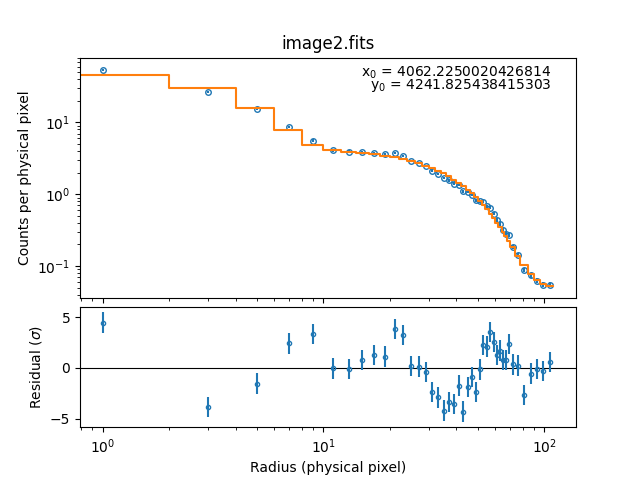### Figure 5: Residuals (in counts) about the fit

The addition of the core component produces a much better fit to the source emission. Note however, that this is a radial profile, so image_resid should still be used to look for differences that may be hidden by the radial averaging.

The group_counts option was used to change the radial binning; after calculating the bins using the default values—e.g. the same as used to create the earlier plots—the data is grouped together until each new radial group contains at least 200 counts. Since the source emission is high in the core regions this only affects the profile at large radii in this example. The 2D fit result is not affected by the grouping scheme set in in prof_fit; the grouping scheme in the function is just to rebin the generated profile that is plotted.

sherpa> prof_fit_delchi(model=src, group_counts=200)


### Figure 6: Residuals (normalized by the error) about the fit### Figure 6: Residuals (normalized by the error) about the fit

This shows a similar plot to Figure 5 but the residuals have been normalized by their errors.

## Using elliptical models

Up until now the models have been circularly-symmetric (i.e. the ellipticity of the components has been zero). We now see what happens if we let the core emission be elliptical:

sherpa> thaw(core)
sherpa> core.ellip = 0.1
sherpa> core.theta = 1
sherpa> fit()
Dataset               = 1
Statistic             = cash
Initial fit statistic = -54684.1
Final fit statistic   = -54814.6 at function evaluation 1178
Data points           = 9171
Degrees of freedom    = 9160
Change in statistic   = 130.486
bgnd.c0        0.194298
src.fwhm       64.4477
src.xpos       4070.62
src.ypos       4251.53
src.ampl       17.0557
core.fwhm      8.30472
core.xpos      4070.74
core.ypos      4249.28
core.ellip     0.331923
core.theta     0.788844
core.ampl      215.863

sherpa> prof_fit(model=core, group_counts=200)Note

Since the range of the theta parameter of the models now defaults to -2π to , rather than having a lower limit of 0, the theta parameter often does not need to be changed before starting a fit.

In earlier versions of CIAO (<4.6) this thread suggested setting

core.theta = 1

before the fit; changing this value before a fit can often be a sensible choice, as with any other parameter, to avoid getting trapped in local minimums.

The resulting plot is shown in Figure 7.

### Figure 7: Elliptical profile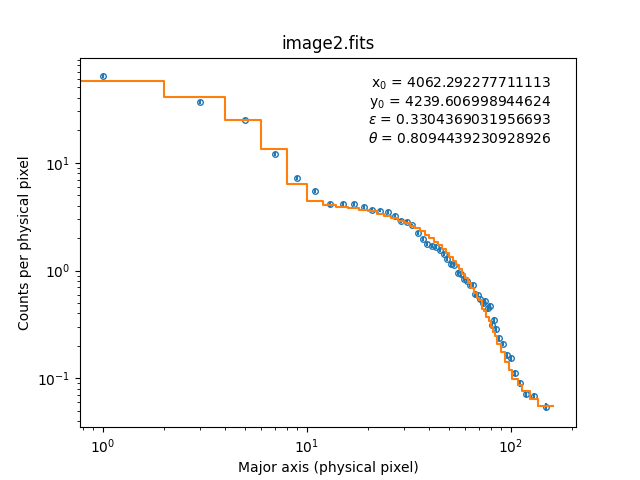### Figure 7: Elliptical profile

Since the model used to define the profile—core—has a non-zero ellipticity then the annuli used to create this profile are elliptical. The values used for the ellipticity and position angle are included in the labels in the top-right of the plot.

You can over-ride any of the component values used to define the profile: in the following we use the position of the core component but ignore the model's ellipticity, and use circular annuli instead. The result is shown in Figure 8.

sherpa> prof_fit(model=core, group_counts=200, ellip=0)


### Figure 8: Over-riding the ellipticity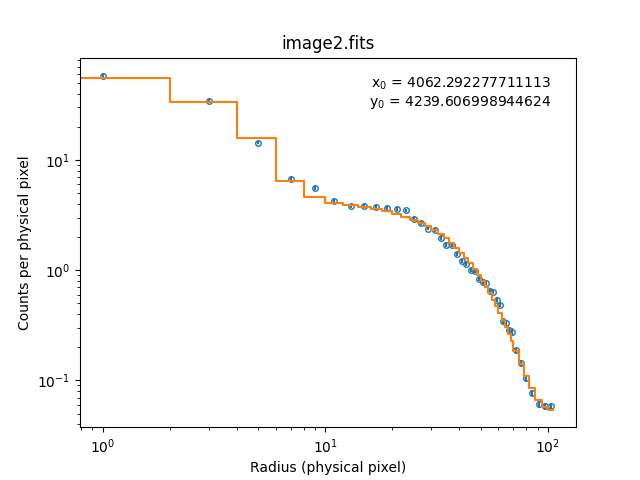### Figure 8: Over-riding the ellipticity

The same profile center is used as in Figure 7 but the ellipticity has been over-ridden, and set to 0, by use of the ellip argument to prof_fit.

## Overlays and data access

In this section we shall highlight some of the other features of the profiles package:

• plot preferences
• plot overlays
• data access

### Plot preferences

The individual plots—such as data, model, resid, and delchi—have preference settings. The current settings can be retrieved with the get_<type>_prof_prefs command: for example

sherpa> print(get_data_prof_prefs())
{'yerrorbars': True, 'ecolor': None, 'capsize': None, 'barsabove': False, 'xlog': True, 'ylog': True, 'linestyle': '', 'linecolor': None, 'color': None, 'marker': 'o', 'markerfacecolor': 'none', 'markersize': 4}


These values can be changed, and new ones added, either by saying

sherpa> get_data_prof_prefs()["markercolor"] = "green"


or by assigning a variable to the return value of the call and then changing the variable:

sherpa> p = get_data_prof_prefs()
sherpa> p["markercolor"] = "green"
sherpa> p["ecolor"] = "blue"


A full list of the preferences can be found in the following ahelp pages:

### Overlaying data

The overplot argument can be used to overlay data on an existing plot. The commands

sherpa> prof_data(model=core)
sherpa> prof_model(model=core, overplot=True)


produce a plot similar to plot_fit. This capability extends to plot_fit, so you could say

sherpa> prof_fit(model=core)
sherpa> prof_fit(2, model=core, overplot=True)


to overplot the data and model from dataset 2 onto the plot from the default dataset.

### Accessing the plot data

For the basic plot types—e.g. data, model, source, resid, delchi, and fit—there are corresponding get_<type>_prof() calls which return objects which contain the data used to create the plots. These routines can be called without having to call the corresponding prof_<type>() routine first.

## Scripting It

The commands used to run this thread may be saved in a text file such as fit.py, which can then be executed as a script: %run -i fit.py. Alternatively, the Sherpa session can be saved to a binary file with the save command (restored with the restore command), or to an editable ASCII file with save_all.

The Sherpa script command may be used to save everything typed on the command line in a Sherpa session:

sherpa> script(filename="sherpa.log", clobber=False)


(Note that restoring a Sherpa session from such a file could be problematic since it may include syntax errors, unwanted fitting trials, et cetera.)

## Summary

This thread showed you how to use the plotting routines from the sherpa_contrib.profiles package. These routines allow you to view a radial plot of your two-dimensional data, model, fit, or residuals; this allows you to inspect the fit parameters so that you start closer to the best-fit solution or to visually inspect the fit results. Since they radially average the results you should always also look at the image residuals too, using the image_fit command.

Both sets of routines can be loaded in one go using the sherpa_contrib module.